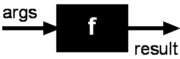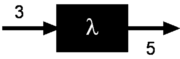#Boost C++ Libraries

...one of the most highly regarded and expertly designed C++ library projects in the world.

## Basics

Almost everything is a function in the Phoenix library that can be evaluated as `f(a1, a2, ..., a/n/)`, where n is the function's arity, or number of arguments that the function expects. Operators are also functions. For example, `a + b` is just a function with arity == 2 (or binary). ```a + b``` is the same as `add(a, b)`, ```a + b + c``` is the same as ```add(add(a, b), c)```.

NoteAmusingly, functions may even return functions. We shall see what this means in a short while.

#### Partial Function Application

Think of a function as a black box. You pass arguments and it returns something back. The figure below depicts the typical scenario.A fully evaluated function is one in which all the arguments are given. All functions in plain C++ are fully evaluated. When you call the `sin(x)` function, you have to pass a number x. The function will return a result in return: the sin of x. When you call the `add(x, y)` function, you have to pass two numbers x and y. The function will return the sum of the two numbers. The figure below is a fully evaluated `add` function.A partially applied function, on the other hand, is one in which not all the arguments are supplied. If we are able to partially apply the function `add` above, we may pass only the first argument. In doing so, the function does not have all the required information it needs to perform its task to compute and return a result. What it returns instead is another function, a lambda function. Unlike the original `add` function which has an arity of 2, the resulting lambda function has an arity of 1. Why? because we already supplied part of the input: `2`Now, when we shove in a number into our lambda function, it will return 2 plus whatever we pass in. The lambda function essentially remembers 1) the original function, `add`, and 2) the partial input, 2. The figure below illustrates a case where we pass 3 to our lambda function, which then returns 5:Obviously, partially applying the `add` function, as we see above, cannot be done directly in C++ where we are expected to supply all the arguments that a function expects. That's where the Phoenix library comes in. The library provides the facilities to do partial function application. And even more, with Phoenix, these resulting functions won't be black boxes anymore.

#### STL and higher order functions

So, what's all the fuss? What makes partial function application so useful? Recall our original example in the previous section:

```std::find_if(c.begin(), c.end(), arg1 % 2 == 1)
```

The expression ```arg1 % 2 == 1``` evaluates to a lambda function. `arg1` is a placeholder for an argument to be supplied later. Hence, since there's only one unsupplied argument, the lambda function has an arity 1. It just so happens that `find_if` supplies the unsupplied argument as it loops from `c.begin()` to `c.end()`.

NoteHigher order functions are functions which can take other functions as arguments, and may also return functions as results. Higher order functions are functions that are treated like any other objects and can be used as arguments and return values from functions.

#### Lazy Evaluation

In Phoenix, to put it more accurately, function evaluation has two stages:

1. Partial application
2. Final evaluation

The first stage is handled by a set of generator functions. These are your front ends (in the client's perspective). These generators create (through partial function application), higher order functions that can be passed on just like any other function pointer or function object. The second stage, the actual function call, can be invoked or executed anytime in the future, or not at all; hence "lazy".

If we look more closely, the first step involves partial function application:

```arg1 % 2 == 1
```

The second step is the actual function invocation (done inside the `find_if` function. These are the back-ends (often, the final invocation is never actually seen by the client). In our example, the `find_if`, if we take a look inside, we'll see something like:

```template <class InputIterator, class Predicate>
InputIterator
find_if(InputIterator first, InputIterator last, Predicate pred)
{
while (first != last && !pred(*first))  // <--- The lambda function is called here
++first;                            //      passing in *first
return first;
}
```

Again, typically, we, as clients, see only the first step. However, in this document and in the examples and tests provided, don't be surprised to see the first and second steps juxtaposed in order to illustrate the complete semantics of Phoenix expressions. Examples:

```int x = 1;
int y = 2;

std::cout << (arg1 % 2 == 1)(x) << std::endl; // prints 1 or true
std::cout << (arg1 % 2 == 1)(y) << std::endl; // prints 0 or false
```

#### Forwarding Function Problem

Usually, we, as clients, write the call-back functions while libraries (such as STL) provide the callee (e.g. `find_if`). In case the role is reversed, e.g. if you have to write an STL algorithm that takes in a predicate, or develop a GUI library that accepts event handlers, you have to be aware of a little known problem in C++ called the "Forwarding Function Problem".

Look again at the code above:

```(arg1 % 2 == 1)(x)
```

Notice that, in the second-stage (the final evaluation), we used a variable `x`.

In Phoenix we emulated perfect forwarding through preprocessor macros generating code to allow const and non-const references.

We generate these second-stage overloads for Phoenix expression up to `BOOST_PHOENIX_PERFECT_FORWARD_LIMIT`

NoteYou can set `BOOST_PHOENIX_PERFECT_FORWARD_LIMIT`, the predefined maximum perfect forward arguments an actor can take. By default, `BOOST_PHOENIX_PERFECT_FORWARDLIMIT` is set to 3.

#### Polymorphic Functions

Unless otherwise noted, Phoenix generated functions are fully polymorphic. For instance, the `add` example above can apply to integers, floating points, user defined complex numbers or even strings. Example:

```std::string h("Hello");
char const* w = " World";
std::string r = add(arg1, arg2)(h, w);
```

evaluates to ```std::string("Hello World")```. The observant reader might notice that this function call in fact takes in heterogeneous arguments where `arg1` is of type `std::string` and `arg2` is of type `char const*`. `add` still works because the C++ standard library allows the expression `a + b` where `a` is a `std::string` and `b` is a ```char const*```.